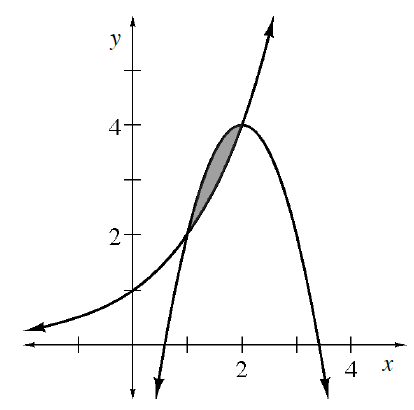### Home > CALC3RD > Chapter Ch5 > Lesson 5.2.3 > Problem5-85

5-85.

Use your calculator to estimate the shaded area shown between the graphs of $y = 4 – 2\left(x – 2\right)^{2}$ and $y = 2^{x}$.Identify where the functions intersect, as well as which function is 'greater' (has higher $y$-values) than the other.

$\text{Integrate}: \int_{\text{intersection }A}^{\text{intersection }B}[\text{top function}]-[\text{bottom function}]dx$